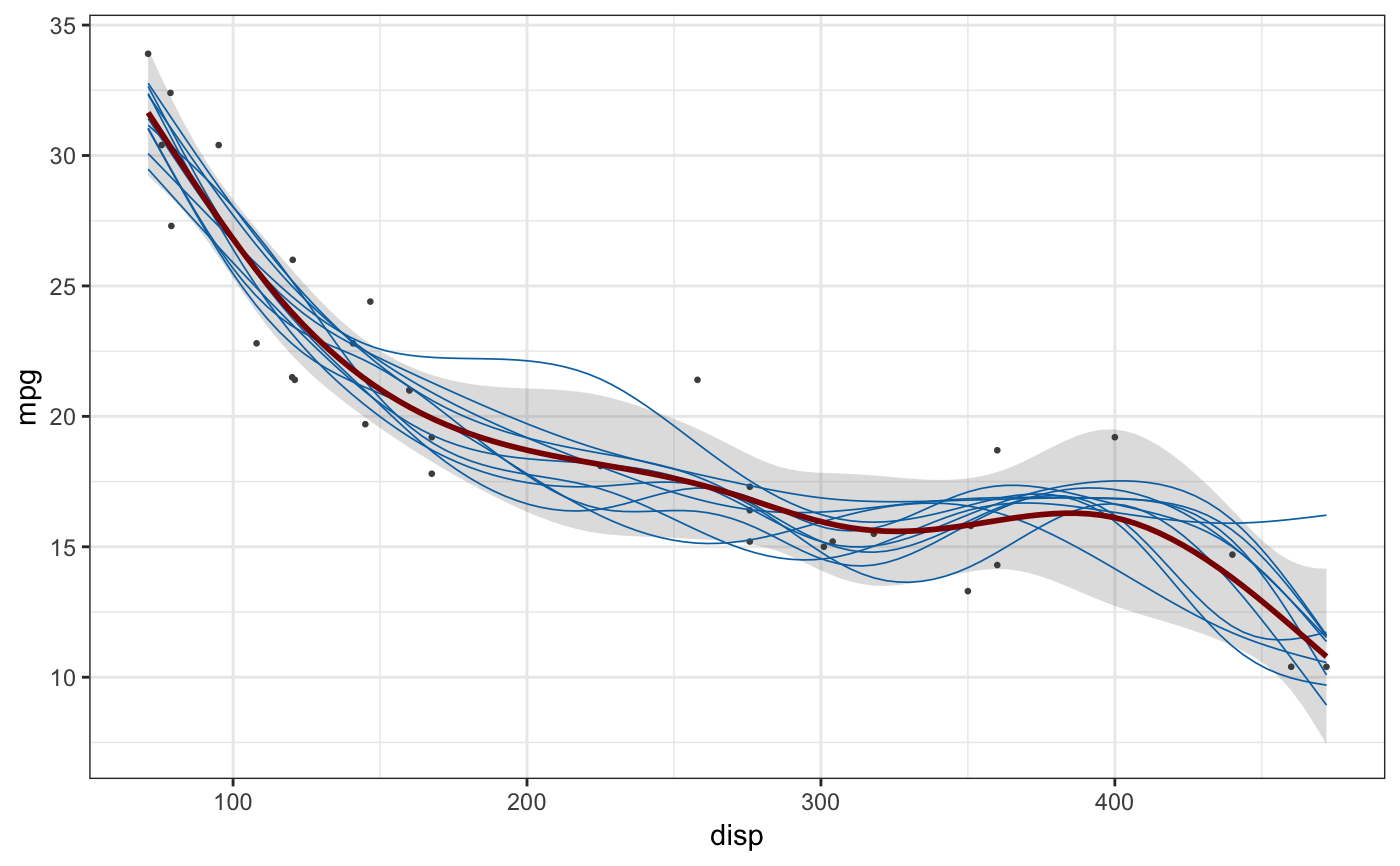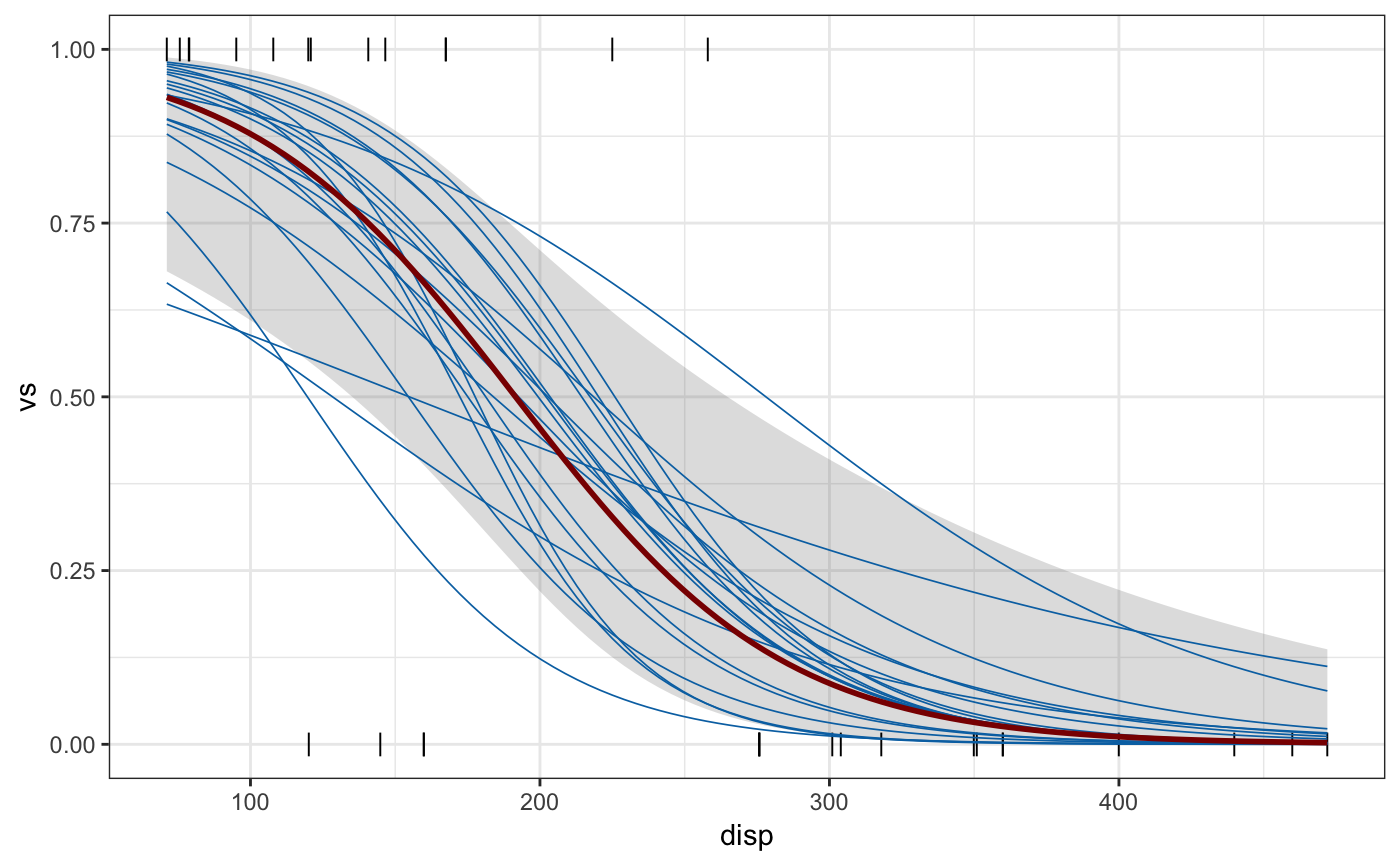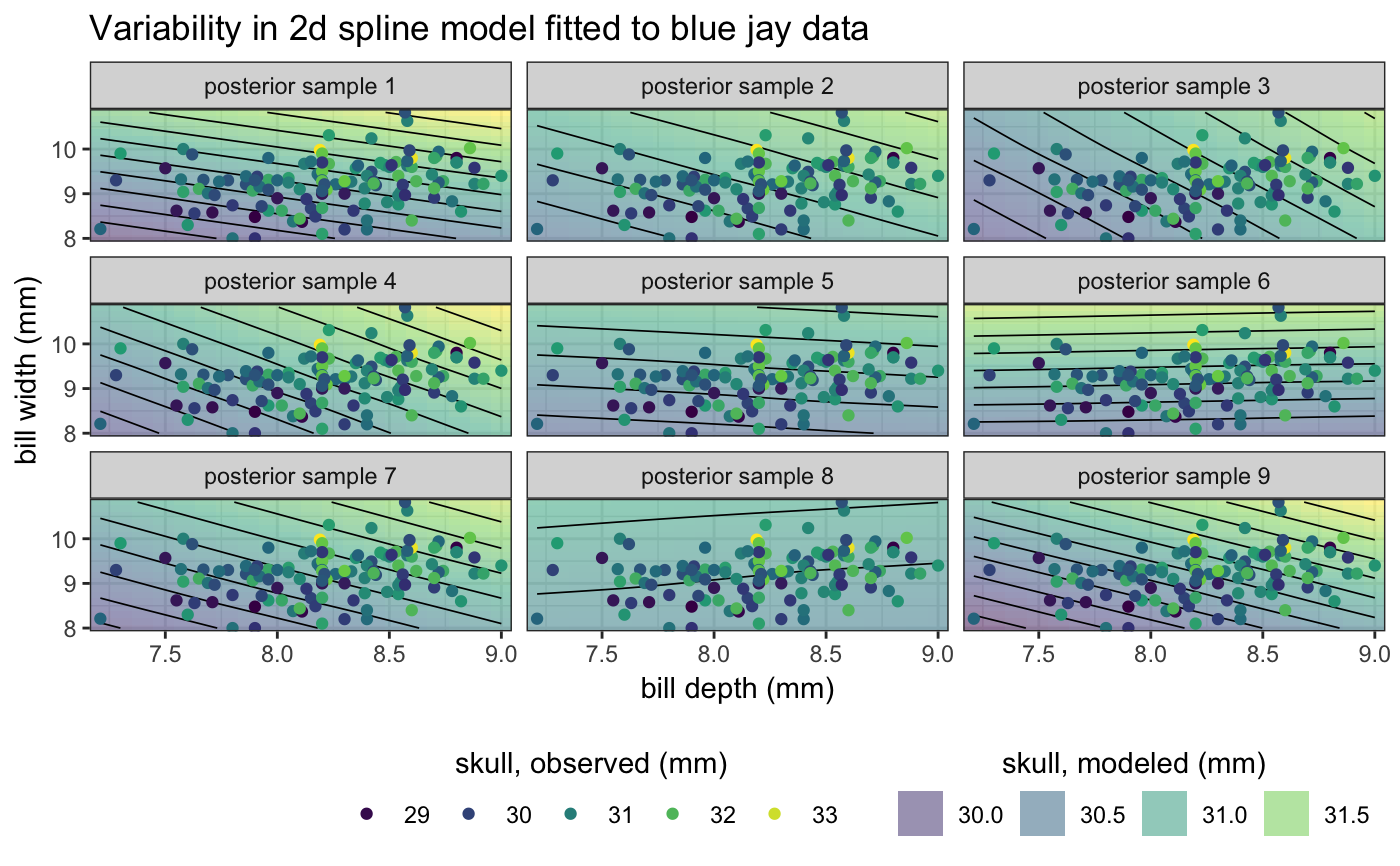Sample outcomes from a fitted model (via sample_outcomes()) or calculate a confidence band for the fitted model (via confidence_band()). Currently, sampling of fitted models is only implemented for generalized additive models fitted with mgcv::gam().

sample_outcomes(model, newdata, times = 20, key = ".draw", ...)

# S3 method for gam
sample_outcomes(model, newdata, times = 20,
key = ".draw", unconditional = FALSE, ...)

confidence_band(model, newdata, level = 0.95, ...)

# S3 method for gam
confidence_band(model, newdata, level = 0.95,
unconditional = FALSE, ...)

## Arguments

model The fitted model to use for generating outcomes or calculating a confidence band. A data frame containing the values of the model covariates at which outcomes or confidence values should be calculated, as in predict.gam(). Number of times outcomes should be drawn. Character vector holding the name of the column indicating separate outcome draws. Default is .draw. Other arguments. Bool indicating whether the smoothing parameter uncertainty corrected covariance matrix is used (TRUE) or not (FALSE, the default), as in predict.gam(). A choice of unconditional = TRUE will generally require the gam model to be fit by REML (method = "REML" in the gam() call). Confidence level. Default is 95%.

## Examples

library(mgcv)#> Loading required package: nlme#>
#> Attaching package: ‘nlme’#> The following object is masked from ‘package:dplyr’:
#>
#>     collapse#> This is mgcv 1.8-24. For overview type 'help("mgcv-package")'.library(ggplot2)

# gam spline fit
fit <- gam(mpg ~ s(disp), data = mtcars, method = "REML")

newdata <- data.frame(
disp = seq(
min(mtcars$disp), max(mtcars$disp),
length.out = 100
)
)

sample_df <- sample_outcomes(fit, newdata, 10, unconditional = TRUE)
conf <- confidence_band(fit, newdata, unconditional = TRUE)
ggplot(mtcars, aes(disp, mpg)) +
geom_ribbon(data = conf, aes(ymin = lo, ymax = hi), fill="#80808040", color = NA) +
geom_point(color = "grey30", size = 0.5) +
geom_line(data = sample_df, aes(group = .draw), color = "#0072B2", size = 0.3) +
geom_line(data = conf, size = 1, color = "darkred") +
theme_bw()# logistic regression fit
fit <- gam(vs ~ disp, data = mtcars, family = "binomial")

sample_df <- sample_outcomes(fit, newdata, 20)
conf <- confidence_band(fit, newdata)
ggplot(mtcars, aes(disp, vs)) +
geom_ribbon(data = conf, aes(ymin = lo, ymax = hi), fill="#80808040", color = NA) +
geom_point(color = "black", size = 3, shape = "|") +
geom_line(data = sample_df, aes(group = .draw), color = "#0072B2", size = 0.3) +
geom_line(data = conf, size = 1, color = "darkred") +
theme_bw()# example in 2D
data(BlueJays, package = "Stat2Data")

fit <- gam(Skull ~ s(BillDepth, BillWidth), data = BlueJays, method = "REML")

newdata <- expand.grid(
BillWidth = seq(min(BlueJays$BillWidth), max(BlueJays$BillWidth), length.out = 20),
BillDepth = seq(min(BlueJays$BillDepth), max(BlueJays$BillDepth), length.out = 20)
)

sample_df <- sample_outcomes(fit, newdata, 9, unconditional = TRUE)
ggplot(BlueJays, aes(BillDepth, BillWidth)) +
geom_tile(data = sample_df, aes(fill = Skull), alpha = 0.5, color = NA) +
geom_contour(data = sample_df, aes(z = Skull), color = "black", size = 0.3, binwidth = 0.2) +
geom_point(aes(color = Skull), size = 1.5) +
scale_fill_viridis_c(
name = "skull, modeled (mm)",
option = "D",
guide = guide_legend(
title.position = "top",
order = 2,
override.aes = list(alpha = 0.5)
)
) +
scale_color_viridis_c(
name = "skull, observed (mm)",
option = "D",
guide = guide_legend(title.position = "top", order = 1)
) +
coord_cartesian(expand = FALSE) +
xlab("bill depth (mm)") +
ylab("bill width (mm)") +
ggtitle("Variability in 2d spline model fitted to blue jay data") +
facet_wrap(~.draw, labeller = as_labeller(function(x) paste0("posterior sample ", x))) +
theme_bw() +
theme(
legend.direction = "horizontal",
legend.position = "bottom",
legend.justification = "right",
legend.title.align = 0.5
)